# Electronics and Communication Engineering - Exam Questions Papers

26.

The skin-depth of copper at a frequency of 3 GHz is 1 micron. At 12 GHz, for a non-magnetic conductor whose conductivity is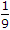times of copper, the skin depth would be :

 A. 9 x 4 μm B.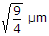C.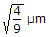D.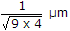Explanation: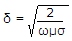.

27.

If the z-transform of x(n) is given by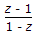then the filter it represents is __________ .

 A. low pass B. high pass C. band pass D. all pass

Explanation: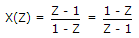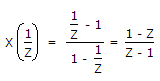i.e. we can see that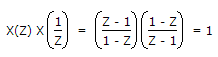According to the property, the filter is an all-pass filter.

28.

The system of linear equations 4x + 2y = 1 , 2x + y = 6 has

 A. a unique solution B. no solution C. an infinite number of solutions D. exactly two distinct solutions

Explanation:

4x + 1y = 7 → (1)

2x + y = 6 → (2)

(1) can be written as 2x + y = 3.5 → (3)

Now LHS of equations (2) and (3) are same but RHS is different which is not possible. Hence no solution.

29.

Which of the following periodic waveforms will have only odd harmonics of sinusoidal waveform?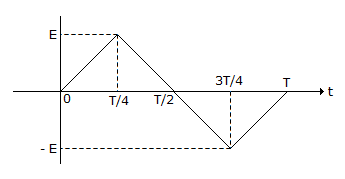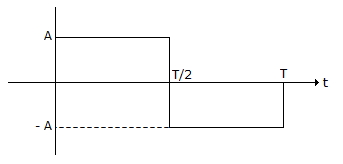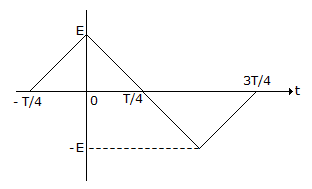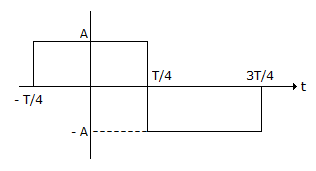Select the correct option using the codes given below Codes:

 A. 1 and 2 B. 1 and 3 C. 1 and 4 D. 2 and 4

Explanation:

The conditions for a signal s(t) to have odd harmonics of sinusoidal waveforms alone are given by

(i)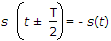, where T is the period and

(ii) s(t) = - s(t) [i.e., odd function]

The waveforms (1) and (2) satisfy condition (b).

30.

A control system is as shown in given figure The maximum value of gain K for which the system is stable is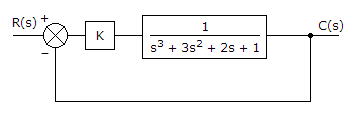A. more than 5 B. equal to 5 C. less than 1 D. less than 5

Explanation:

Characteristic Equation is 1 + GH = 0

1 +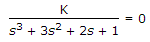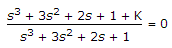or s3 + 3s2 + 2s + (1 + K) = 0

Hurwitz criterion can be applied

s3 1 2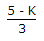and (1 + K) both should be + ve

s2 3 1 + K

s1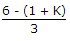0 K should be more than - 1 and less than 5

s0 1 + K.

#### Current Affairs 2022

Interview Questions and Answers